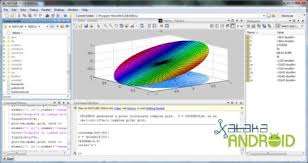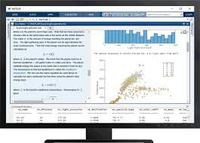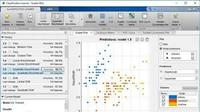MATLAB

17.11.2020

Matlab is an abbreviation of matrix laboratory. Matlab is a high performance language of technical computing. It is a product of MathWorks. MathWorks was founded by Cleve Moler, Jack Little and Steve Bangert in 1984. Matlab was originally enhanced by control design engineers. It quickly spread to signal processing and image processing areas. Now it is extensively used in education in teaching linear algebra and numerical techniques.

What is Matlab?

First of all, it's important to define what MATLAB is. For some, it is software, a tool, for others a language.

In fact, it's a bit of all of that.

when we talk about MATLAB software, we are referring to the tool we are using, the user interface;

when we speak of the MATLAB language, we designate the specific syntax that we implement in this tool.

The name MATLAB comes from the English MATrix LABoratory. A literal translation leads us to see MATLAB as a laboratory for manipulating matrices. We will come back to this point, which is a fundamental element of the MATLAB language: most of the functions defined in MATLAB are defined for matrix quantities, and by extension, for tabulated data.

MATLAB includes many functions, for calculating or processing data, display, plotting curves, solving systems and algorithms for numerical calculations in the broad sense of the term.

All these functions are defined by default in MATLAB in a specific programming language which we will call ... MATLAB!And if as standard, MATLAB offers functions covering all of these areas, if your developments require the implementation of very advanced programs, there are more specific functions grouped together in TOOLBOXes (which can be translated as "box tools ") . These toolboxes are obviously useful, even necessary, paid extensions, including functions dedicated to these areas, for professional development.

We can cite the extensions:

• OPTIMIZATION for optimization;
• IMAGE PROCESSING for image processing.

For the novice user, these toolboxes are not necessary at first, but it is important to know that when the time comes, if the need arises, you will have the possibility to use these toolboxes, and their functional richness.In addition, rather quickly we will see that it is possible to enrich the possibilities of MATLAB by developing its own functions, with the language MATLAB. The syntax of this language is accessible to beginners, because everything is done to overcome the constraints imposed by most programming languages:

- no production chains

- editing

- compilation

- execution

- no need to declare and type functions

We will however see that the notion of type exists in this language as in the others, and that if this notion is hidden from the beginner user, it can be useful to take it into account ...

Likewise, if it is possible to avoid the compilation phase, this possibility which facilitates the use of this language for the beginner, it can prove to be an obstacle to the use of MATLAB for advanced developments, which would then ask to use another more efficient language.

MATLAB, like any interpreted language, offers overall lower performance than other compiled languages.

However, it must be recognized that the performance of the MATLAB interpreter improves from version to version, which means that in many cases, the computation times offered today are quite honorable.

Finally, note that MATLAB can be run on the most common platforms: linux, mac os X or windows. The lack of compilation makes programs written in scripted form inherently portable: if you write a program using MATLAB on a given platform, you can distribute your code which can be "interpreted" by a user working on a platform. other platform.

Matlab characteristics

This language includes many predefined functions for matrix calculation, but not only. As a result, the fields of application are extremely varied, and one can quote for example:

• numerical computation in the body of reals or complexes
• calculating probabilities or statistics
• integral calculus or derivation
• signal processing
• optimization
• image processing
• automatism

• Implement and test your algorithms easily
• Develop the computational codes easily
• Debug easily
• Use a large database of built in algorithms
• Process still images and create simulation videos easily

• Interpreted language
• Cost

Matlab in Biomedical Engineering

Matlab has tools for almost any field of computing engineering.

You can use it for image analysis, signal analysis, projects modelling, machine learning development, applications development and so on.

Therefore, you can use it for any of the the healthcare applications,  it all depends on the application you make of it.My experience with Matlab

As said before, Matlab provides many tools for biomedical engineers. Personally, Matlab is the environment I have used the most during my career and and after it.

From the moment the basics of `language programming where completly integrated. I have used it to analyse medical images, signal images, applications for biomedical projects when I was at the hospital. I haven't used the machine learning tools yet because I mainly use Python at work.

My opinion is that, except if you know another particular programming language is better, it is a very good option to develop the program you are willing to,  thanks to its many advantages.

Mathworks

MathWorks is an American privately held corporation that specializes in mathematical computing software. Its major products include MATLAB and Simulink, which support data analysis and simulation.